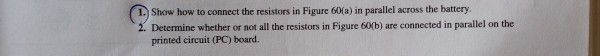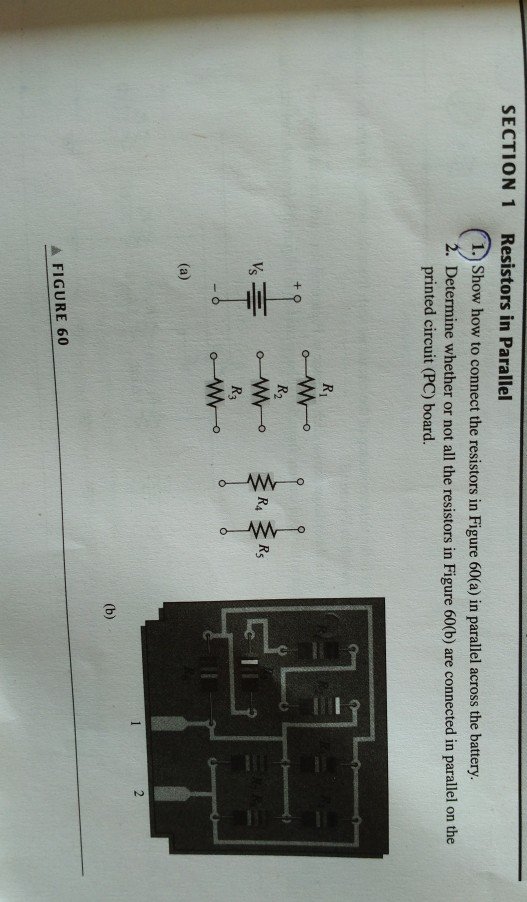# Question 1. Show how to connect the resistors in Figure 60(a) in parallel across the battery 2. Determine whether or not all the resistors in Figure 60b) are connected in parallel on the printed circuit (PC) board. SECTION 1 Resistors in Parallel 1. Show how to connect the resistors in Figure 60(a) in parallel across the battery. 2. Determine whether or not all the resistors in Figure 60(b) are connected in parallel on the printed circuit (PC) board. R WO R2 Vs R4 Rg Wo R3 WO 2 (b) A FIGURE 608DZHSP The Asker · Electrical EngineeringTranscribed Image Text: 1. Show how to connect the resistors in Figure 60(a) in parallel across the battery 2. Determine whether or not all the resistors in Figure 60b) are connected in parallel on the printed circuit (PC) board. SECTION 1 Resistors in Parallel 1. Show how to connect the resistors in Figure 60(a) in parallel across the battery. 2. Determine whether or not all the resistors in Figure 60(b) are connected in parallel on the printed circuit (PC) board. R WO R2 Vs R4 Rg Wo R3 WO 2 (b) A FIGURE 60
More
Transcribed Image Text: 1. Show how to connect the resistors in Figure 60(a) in parallel across the battery 2. Determine whether or not all the resistors in Figure 60b) are connected in parallel on the printed circuit (PC) board. SECTION 1 Resistors in Parallel 1. Show how to connect the resistors in Figure 60(a) in parallel across the battery. 2. Determine whether or not all the resistors in Figure 60(b) are connected in parallel on the printed circuit (PC) board. R WO R2 Vs R4 Rg Wo R3 WO 2 (b) A FIGURE 60